# How Does a Temperature Sensor Work

Temperature sensors are a device which measures the temperature using the electrical signals. Let's learn how a sensor works and how the temperature changes to it accordingly.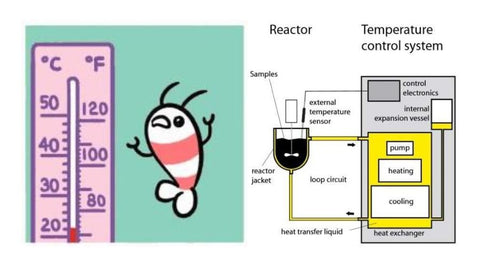When we have a fever we visit a doctor and the doctor checks our temperature using a thermometer. Based on the temperature reading he gives the necessary further

instructions to us. If the reading is too high or in the abnormal range he might give us a medicine prescription.

Depending upon the temperature reading different action is taken by the doctor. Similarly to detect abnormal temperature readings to prevent hazards in various industries (gas boiler, furnace temperature measurement), health care( patient
temperature monitoring), space applications(temperature measurement in rockets), etc it is of cardinal significance to monitor the temperature.

## What is a temperature sensor and how does it work?

The temperature sensor is a device that measures the hotness or coldness and transforms it into an output quantity which is an electrical signal.

## Working principle of temperature sensor

The working of the temperature sensor is dependent on the technology through
which it's manufactured. So depending on the technology used temperature sensors can be classified into contact type and non-contact type.

## Classification of Temperature Sensor

### 1. Contact type temperature sensors

In order to measure temperature, the surface of the sensing element of the temperature sensor needs to be in physical contact with the surface of the object. Following are the different types:

#### a. Thermistor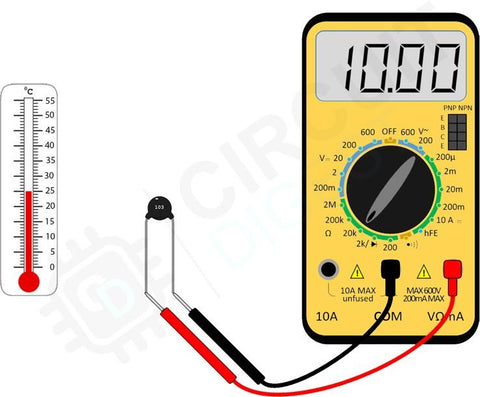A thermistor is a temperature sensor that is made up of semiconductors ( resistance greater than conducting materials but less than insulators) such as metallic oxides squeezed into wafers and then cut to chip size(disc or another shape). Based on the materials used to manufacture their resistance temperature curve varies. So, the thermistor is a temperature sensor whose resistance varies as the temperature is changed.

They can be classified into two classes

• Negative Temperature coefficient (NTC)

Here as temperature increases, the resistance of the conductor/sensor decreases. As shown in the above figure to identify whether the given sensor is NTC or PTC we connect it to a multimeter with a knob switched for measuring resistance. When we supply heat to the sensor, temperature increases, and the resistance reading on the multimeter decreases. Thus, we can say that the given sensor is NC.

• Positive temperature coefficient (PTC)

Here as temperature increases, the resistance of the conductor/sensor increases.

Note:

𝑅 = 𝑅𝑟𝑒𝑓[1 + α(𝑇 − 𝑇𝑟𝑒𝑓)]

𝑅 = 𝐶𝑜𝑛𝑑𝑢𝑐𝑡𝑜𝑟 𝑟𝑒𝑠𝑖𝑠𝑡𝑎𝑛𝑐𝑡 𝑎𝑡 𝑡𝑒𝑚𝑝𝑒𝑟𝑎𝑡𝑢𝑟𝑒 '𝑇'

𝑅𝑟𝑒𝑓 = 𝐶𝑜𝑛𝑑𝑢𝑐𝑡𝑜𝑟 𝑟𝑒𝑠𝑖𝑠𝑡𝑎𝑛𝑐𝑒 𝑎𝑡 𝑟𝑒𝑓𝑒𝑟𝑒𝑛𝑐𝑒 𝑡𝑒𝑚𝑝𝑒𝑟𝑎𝑡𝑢𝑟𝑒 𝑇𝑟𝑒𝑓, 𝑢𝑠𝑢𝑎𝑙𝑙𝑦 20℃ 𝑏𝑢𝑡 𝑠𝑜𝑚𝑒𝑡𝑖𝑚𝑒𝑠 0℃

α = 𝑇𝑒𝑚𝑝𝑒𝑟𝑎𝑡𝑢𝑟𝑒 𝑐𝑜𝑒𝑓𝑓𝑖𝑐𝑖𝑒𝑛𝑡 𝑜𝑓 𝑟𝑒𝑠𝑖𝑠𝑡𝑎𝑛𝑐𝑒 𝑓𝑜𝑟 𝑐𝑜𝑛𝑑𝑢𝑐𝑡𝑜𝑟 𝑚𝑎𝑡𝑒𝑟𝑖𝑎𝑙

𝑇 = 𝐶𝑜𝑛𝑑𝑢𝑐𝑡𝑜𝑟 𝑡𝑒𝑚𝑝𝑒𝑟𝑎𝑡𝑢𝑟𝑒 𝑖𝑛 𝑑𝑒𝑔𝑟𝑒𝑒 𝑐𝑒𝑙𝑠𝑖𝑢𝑠

𝑇𝑟𝑒𝑓 = 𝑅𝑒𝑓𝑒𝑟𝑒𝑛𝑐𝑒 𝑡𝑒𝑚𝑝𝑒𝑟𝑎𝑡𝑢𝑟𝑒 𝑓𝑜𝑟 𝑤ℎ𝑖𝑐ℎ α 𝑖𝑠 𝑔𝑖𝑣𝑒𝑛 𝑓𝑜𝑟 𝑡ℎ𝑒 𝑐𝑜𝑛𝑑𝑢𝑐𝑡𝑜𝑟

The above formula is used to calculate the resistance if the temperature is given and vice versa. While interfacing this sensor with the microcontroller we use the above formula to find the temperature.

When α(temperature coefficient) is positive it means the temperature sensor is PTC

and when α is negative it means the temperature sensor is NTC. This method
calculates the temperature by assuming a linear relationship between temperature and resistance.

Application

In automotive industries to measure the temperature of the battery in electric cars, refrigerators to monitor the temperature.
Note in DHT11 (digital humidity and temperature sensor), a thermistor is used for temperature measurement. This sensor is very easy to use with Arduino as there exists a DHT library. To understand temperature sensors you can try making a circuit and Arduino code with this sensor. Doing practical implementation will give deeper understanding of how the temperature sensors work.

### 2. RTD(Resistance temperature detector)

RTDs are sensors whose resistance increases with an increase in temperature i.e. temperature
coefficient α is positive.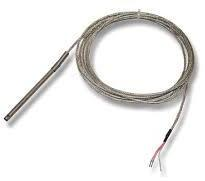RTD’s can be 2-wire, 3-wire, or 4-wire. 4-wire RTDs are the most accurate. Example: PT100(shown in the image, PT1000)

PT100 is a sensor made from platinum material and 100Ω is its resistance at 0℃. Similarly, PT1000 is made from platinum and its resistance is 1000Ω at 0℃.

Their resistance is impeccably linear under a broad operating range and can vary from
-200℃ to 1000℃. They give precise and accurate output but they can be expensive. Example:
In rockets to detect high-temperature changes, consumer electronics, etc.
Note: RTD’s and thermistors will need an extra circuitry for measurement of temperatures like a voltage divider, Wheatstone bridge, or instrumentation amplifier for accurate measurements, to reduce lead resistance and noise reduction.

### 3. Thermocouple

It is a temperature measuring device with two dissimilar conductors joined together to form a junction. When this junction is subject to heat emf gets generated across the end terminals (called Seebeck’s effect). This emf generated is used to calculate the temperature at the junction. The below picture shows a K-type thermocouple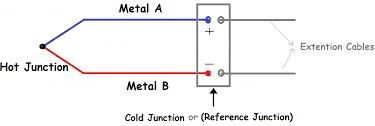The below image shows different thermocouple types and their temperature range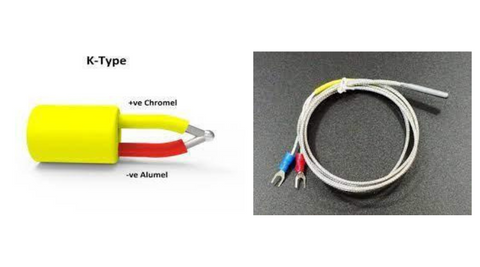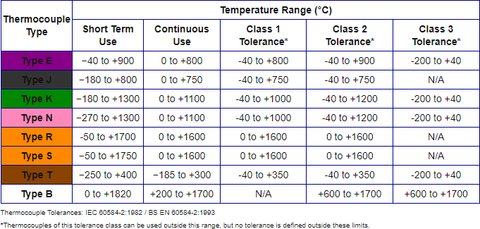Applications

1. Used in home appliances, rockets, etc. Advantages:
2. For very high-temperature applications, they have fast response time, etc Disadvantages:
3. Non-linearity, very less stability.

### 4. Thermostat

It consists of a bimetallic strip ( strip made up of two different metals of different coefficients of expansion) fixed at one end and free to move at the other as shown in the below image. When the strip is subjected to heat it moves/bends towards the metal which has a low coefficient of expansion. So this bend moves the pointer and gives the temperature reading.Applications
Used in heaters, ovens, air conditioners, etc.

### 5. Sensor ICs

The advantage of these types of sensor ICs is that they consist of a temperature sensor, Analog to digital converter, and can be directly connected to the microcontroller.
The range of operation is about -55℃ to +150℃.
Example: LM35 AND DS1621 ( digital temperature sensor IC).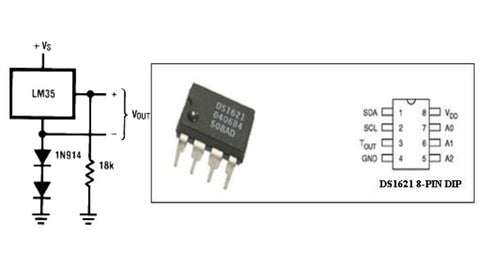### 2. Non-contact type temperature sensorsYou might have come across these types of temperature sensor devices where we get the temperature reading just by pulling the trigger/button of the temperature scanner. So here what happens is the sensor measures the temperature with the help of radiations emitted by the source.All the objects have atoms moving in them (since the objects are not at absolute zero temperature). How fast these atoms move is dependent on the temperature of the object. When these atoms move they emit energy in the form of infrared radiation.
We cannot see this radiation because the frequency of these radiations doesn't lie in the visible light band though we can feel this infrared radiation in the form of heat.
For example, when we go near a bonfire we can feel the heat. This is due to the infrared radiations emitted by the fire.
So, any object which is above absolute zero temperature emits radiations as a function of temperature. This helps us to calculate the temperature by analyzing the light emitted by an object.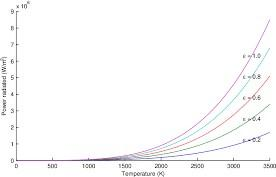## Stefan-Boltzmann Law

Net radiated power emitted from a surface is proportional to the fourth power of its absolute temperature.

𝑃 = σ𝑒𝐴𝑇^4

𝑃 = 𝑁𝑒𝑡 𝑟𝑎𝑑𝑖𝑎𝑡𝑒𝑑 𝑝𝑜𝑤𝑒𝑟, 𝑒 = 𝐸𝑚𝑖𝑠𝑠𝑖𝑣𝑖𝑡𝑦, 𝐴 = 𝑅𝑎𝑑𝑖𝑎𝑡𝑖𝑛𝑔 𝑎𝑟𝑒𝑎, 𝑇 = 𝑇𝑒𝑚𝑝𝑒𝑟𝑎𝑡𝑢𝑟𝑒 𝑜𝑓 𝑟𝑎𝑑𝑖𝑎𝑡𝑜𝑟 σ = 𝑆𝑡𝑒𝑓𝑎𝑛 − 𝐵𝑜𝑙𝑡𝑧𝑚𝑎𝑛𝑛 𝑐𝑜𝑛𝑠𝑡𝑎𝑛𝑡Examples: Thermopile, thermal imager, IR pyrometer, etc. As shown in the above image.

## Conclusion

The temperature sensor is a device that measures the hotness or coldness and transforms it into an output quantity which is an electrical signal.
The working of the temperature sensor is dependent on the technology through which it's manufactured